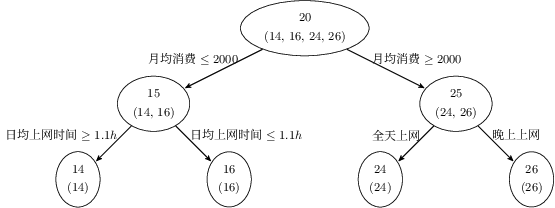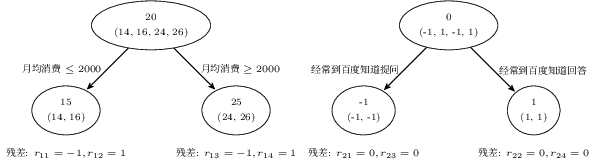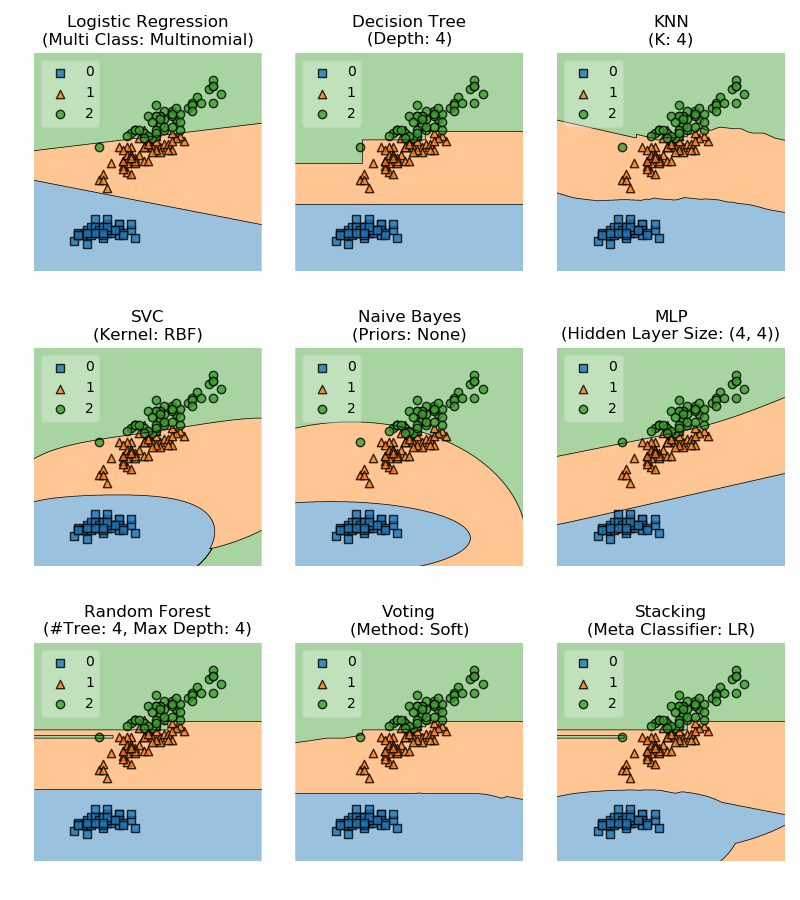# 集成学习算法 (Ensemble Learning)

Thomas G. Dietterich 1 2 指出了集成算法在统计，计算和表示上的有效原因：

• 统计上的原因

• 计算上的原因

• 表示上的原因

# Bagging

Bagging (Boostrap Aggregating) 是由 Breiman 于 1996 年提出 5，基本思想如下：

1. 每次采用有放回的抽样从训练集中取出 $n$ 个训练样本组成新的训练集。
2. 利用新的训练集，训练得到 $M$ 个子模型 $\{h_1, h_2, ..., h_M\}$
3. 对于分类问题，采用投票的方法，得票最多子模型的分类类别为最终的类别；对于回归问题，采用简单的平均方法得到预测值。

Bagging 算法如下所示：

\begin{algorithm}
\caption{Bagging 算法}
\begin{algorithmic}
\REQUIRE \\
学习算法 $L$ \\
子模型个数 $M$ \\
训练数据集 $T = \{(x_1, y_1), (x_2, y_2), ..., (x_N, y_N)\}$
\ENSURE \\
Bagging 算法 $h_f\left(x\right)$
\FUNCTION{Bagging}{$L, M, T$}
\FOR{$m = 1$ \TO $M$}
\STATE $T_m \gets$ boostrap sample from training set $T$
\STATE $h_m \gets L\left(T_m\right)$
\ENDFOR
\STATE $h_f\left(x\right) \gets \text{sign} \left(\sum_{m=1}^{M} h_m\left(x\right)\right)$
\RETURN $h_f\left(x\right)$
\ENDFUNCTION
\end{algorithmic}
\end{algorithm}


$$\lim_{x \to \infty} \left(1 - \dfrac{1}{M}\right)^M = \dfrac{1}{e} \approx 0.368$$

## 随机森林 (Random Forests)

• 数据采样

• 树的构建

1. 由于随机森林引入了样本扰动和特征扰动，从而很大程度上提高了模型的泛化能力，尽可能地避免了过拟合现象的出现。
2. 随机森林可以处理高维数据，无需进行特征选择，在训练过程中可以得出不同特征对模型的重要性程度。
3. 随机森林的每个基分类器采用决策树，方法简单且容易实现。同时每个基分类器之间没有相互依赖关系，整个算法易并行化。

# Boosting

Boosting 是一种提升算法，可以将弱的学习算法提升 (boost) 为强的学习算法。基本思路如下：

1. 利用初始训练样本集训练得到一个基学习器。
2. 提高被基学习器误分的样本的权重，使得那些被错误分类的样本在下一轮训练中可以得到更大的关注，利用调整后的样本训练得到下一个基学习器。
3. 重复上述步骤，直至得到 $M$ 个学习器。
4. 对于分类问题，采用有权重的投票方式；对于回归问题，采用加权平均得到预测值。

$$T = \{\left(x_1, y_1\right), \left(x_2, y_2\right), ..., \left(x_n, y_n\right)\}$$

$$\begin{equation} \begin{split} D_1 =& \left(w_{11}, w_{12}, ..., w_{1n}\right) \\ w_{1i} =& \dfrac{1}{n}, i = 1, 2, ..., n \end{split} \end{equation}$$

$$\alpha_m = \dfrac{1}{2} \ln \dfrac{1 - \epsilon_m}{\epsilon_m}$$

$$\begin{equation} \begin{split} D_{m+1} =& \left(w_{m+1, 1}, w_{m+1, 2}, ..., w_{m+1, n}\right) \\ w_{m+1, i} =& \dfrac{w_{m, i}}{Z_m} \exp \left(-\alpha_m y_i h_m\left(x_i\right)\right) \end{split} \end{equation}$$

$$Z_m = \sum_{i = 1}^{n} w_{m, i} \exp \left(-\alpha_m y_i h_m \left(x_i\right)\right)$$

$$h_f\left(x\right) = \text{sign} \left(\sum_{m=1}^{M} \alpha_m h_m\left(x\right)\right)$$

\begin{algorithm}
\begin{algorithmic}
\REQUIRE \\
学习算法 $L$ \\
子模型个数 $M$ \\
训练数据集 $T = \{(x_1, y_1), (x_2, y_2), ..., (x_N, y_N)\}$
\ENSURE \\
AdaBoost 算法 $h_f\left(x\right)$
\FUNCTION{AdaBoost}{$L, M, T$}
\STATE $D_1\left(x\right) \gets 1 / n$
\FOR{$m = 1$ \TO $M$}
\STATE $T_{sub} \gets$ sample from training set $T$ with weights
\STATE $h_m \gets L\left(T_{sub}\right)$
\STATE $\epsilon_m\gets Error\left(h_m\right)$
\IF{$\epsilon_m > 0.5$}
\BREAK
\ENDIF
\STATE $\alpha_m \gets \dfrac{1}{2} \ln \dfrac{1 - \epsilon_m}{\epsilon_m}$
\STATE $D_{m+1} \gets \dfrac{D_m \exp \left(-\alpha_m y h_m\left(x\right)\right)}{Z_m}$
\ENDFOR
\STATE $h_f\left(x\right) \gets \text{sign} \left(\sum_{m=1}^{M} \alpha_m h_m\left(x\right)\right)$
\RETURN $h_f\left(x\right)$
\ENDFUNCTION
\end{algorithmic}
\end{algorithm}


## GBDT (GBM, GBRT, MART)

GBDT (Gradient Boosting Decision Tree) 是另一种基于 Boosting 思想的集成算法，除此之外 GBDT 还有很多其他的叫法，例如：GBM (Gradient Boosting Machine)，GBRT (Gradient Boosting Regression Tree)，MART (Multiple Additive Regression Tree) 等等。GBDT 算法由 3 个主要概念构成：Gradient Boosting (GB)，Regression Decision Tree (DT 或 RT) 和 Shrinkage。1. $p_1$ ：14 岁高一学生。购物较少，经常问学长问题，预测年龄 $Age = 15 - 1 = 14$
2. $p_2$ ：16 岁高三学生。购物较少，经常回答学弟问题，预测年龄 $Age = 15 + 1 = 16$
3. $p_3$ ：24 岁应届毕业生。购物较多，经常问别人问题，预测年龄 $Age = 25 - 1 = 24$
4. $p_4$ ：26 岁 2 年工作经验员工。购物较多，经常回答别人问题，预测年龄 $Age = 25 + 1 = 26$

\begin{algorithm}
\caption{GBDT 算法}
\begin{algorithmic}
\REQUIRE \\
子模型个数 $M$ \\
训练数据集 $T = \{(x_1, y_1), (x_2, y_2), ..., (x_N, y_N)\}$
\ENSURE \\
GBDT 算法 $h_f\left(x\right)$
\FUNCTION{GBDT}{$M, T$}
\STATE $F_1\left(x\right) \gets \sum_{i = 1}^{N} y_i / N$
\FOR{$m = 1$ \TO $M$}
\STATE $r_m \gets y - F_m \left(x\right)$
\STATE $T_m \gets \left(x, r_m\right)$
\STATE $h_m \gets RegressionTree \left(T_m\right)$
\STATE $\alpha_m \gets \dfrac{\sum_{i = 1}^{N} r_{im} h_m \left(x_i\right)}{\sum_{i = 1}^{N} h_m \left(x_i\right)^2}$
\STATE $F_m \left(x\right) = F_{m-1} \left(x\right) + \alpha_m h_m \left(x\right)$
\ENDFOR
\STATE $h_f\left(x\right) = F_M \left(x\right)$
\RETURN $h_f\left(x\right)$
\ENDFUNCTION
\end{algorithmic}
\end{algorithm}


GBDT 中也应用到了 Shrinkage 的思想，其基本思想可以理解为每一轮利用残差学习得到的回归树仅学习到了一部分知识，因此我们无法完全信任一棵树的结果。Shrinkage 思想认为在新的一轮学习中，不能利用全部残差训练模型，而是仅利用其中一部分，即：

$$r_m = y - s F_m \left(x\right), 0 \leq s \leq 1$$

## XGBoost

XGBoost 是由 Chen 等人 9 提出的一种梯度提升树模型框架。XGBoost 的基本思想同 GBDT 一样，对于一个包含 $n$ 个样本和 $m$ 个特征的数据集 $\mathcal{D} = \left\{\left(\mathbf{x}_i, y_i\right)\right\}$，其中 $\left|\mathcal{D}\right| = n, \mathbf{x}_i \in \mathbb{R}^m, y_i \in \mathbb{R}$，一个集成树模型可以用 $K$ 个加法函数预测输出：

$$\hat{y}_i = \phi \left(\mathbf{x}_i\right) = \sum_{k=1}^{K}{f_k \left(\mathbf{x}_i\right)}, f_k \in \mathcal{F}$$

XGBoost 首先对损失函数进行了改进，添加了 L2 正则项，同时进行了二阶泰勒展开。损失函数表示为：

$$\begin{equation} \begin{split} \mathcal{L} \left(\phi\right) = \sum_{i}{l \left(\hat{y}_i, y_i\right)} + \sum_{k}{\Omega \left(f_k\right)} \\ \text{where} \ \Omega \left(f\right) = \gamma T + \dfrac{1}{2} \lambda \left\| w \right\|^2 \end{split} \end{equation}$$

$$\mathcal{L}^{\left(t\right)} = \sum_{i=1}^{n}{l \left(y_i, \hat{y}_i^{\left(t-1\right)} + f_t \left(\mathbf{x}_i\right)\right)} + \Omega \left(f_t\right)$$

$$\mathcal{L}^{\left(t\right)} \simeq \sum_{i=1}^{n}{\left[l \left(y_i, \hat{y}_i^{\left(t-1\right)}\right) + g_i f_t \left(\mathbf{x}_i\right) + \dfrac{1}{2} h_i f_t^2 \left(\mathbf{x}_i\right)\right]} + \Omega \left(f_t\right)$$

$$\tilde{\mathcal{L}}^{\left(t\right)} = \sum_{i=1}^{n}{\left[ g_i f_t \left(\mathbf{x}_i\right) + \dfrac{1}{2} h_i f_t^2 \left(\mathbf{x}_i\right)\right]} + \Omega \left(f_t\right)$$

$I_j = \left\{i \ | \ q \left(\mathbf{x}_i\right) = j\right\}$ 表示叶子节点 $j$ 的实例集合，上式可重写为：

$$\begin{equation} \begin{split} \tilde{\mathcal{L}}^{\left(t\right)} &= \sum_{i=1}^{n}{\left[ g_i f_t \left(\mathbf{x}_i\right) + \dfrac{1}{2} h_i f_t^2 \left(\mathbf{x}_i\right)\right]} + \gamma T + \dfrac{1}{2} \lambda \sum_{j=1}^{T}{w_j^2} \\ &= \sum_{j=1}^{T}{\left[\left(\sum_{i \in I_j}{g_i}\right) w_j + \dfrac{1}{2} \left(\sum_{i \in I_j}{h_i + \lambda}\right) w_j^2\right]} + \gamma T \end{split} \end{equation}$$

$$w_j^* = - \dfrac{\sum_{i \in I_j}{g_i}}{\sum_{i \in I_j}{h_i} + \lambda}$$

$$\tilde{\mathcal{L}}^{\left(t\right)} \left(q\right) = - \dfrac{1}{2} \sum_{j=1}^{T}{\dfrac{\left(\sum_{i \in I_j}{g_i}\right)^2}{\sum_{i \in I_j}{h_i} + \lambda}} + \gamma T$$

$$\mathcal{L}_{\text{split}} = \dfrac{1}{2} \left[\dfrac{\left(\sum_{i \in I_L}{g_i}\right)^2}{\sum_{i \in I_L}{h_i} + \lambda} + \dfrac{\left(\sum_{i \in I_R}{g_i}\right)^2}{\sum_{i \in I_R}{h_i} + \lambda} - \dfrac{\left(\sum_{i \in I}{g_i}\right)^2}{\sum_{i \in I}{h_i} + \lambda}\right] - \gamma$$

XGBoost 也采用了 Shrinkage 的思想减少每棵树的影响，为后续树模型留下更多的改进空间。同时 XGBoost 也采用了随机森林中的特征下采样 (列采样) 方法用于避免过拟合，同时 XGBoost 也支持样本下采样 (行采样)。XGBoost 在分裂点的查找上也进行了优化，使之能够处理无法将全部数据读入内存的情况，同时能够更好的应对一些由于数据缺失，大量零值和 One-Hot 编码导致的特征稀疏问题。除此之外，XGBoost 在系统实现，包括：并行化，Cache-Aware 加速和数据的核外计算 (Out-of-Core Computation) 等方面也进行了大量优化，相关具体实现请参见论文和 文档

## LightGBM

LightGBM 是由微软研究院的 Ke 等人 10 提出了一种梯度提升树模型框架。之前的 GBDT 模型在查找最优分裂点时需要扫描所有的样本计算信息增益，因此其计算复杂度与样本的数量和特征的数量成正比，这使得在处理大数据量的问题时非常耗时。LightGBM 针对这个问题提出了两个算法：

2. Exclusive Feature Bundling (EFB)

GOSS 的做法是保留具有较大梯度的样本，并从具有较小梯度的样本中随机采样。同时为了补偿对数据分布的影响，在计算信息增益的时候，GOSS 针对梯度较小的样本引入了一个常数乘子。这样就保证了模型更多的关注未得到较好训练的数据，同时又不会对原始数据分布改变过多。整个算法流程如下：

\begin{algorithm}
\caption{GOSS 算法}
\begin{algorithmic}
\INPUT \\
训练数据 $I$ \\
迭代次数 $d$ \\
具有较大梯度数据的采样比例 $a$ \\
具有较小梯度数据的采样比例 $b$ \\
损失函数 $loss$ \\
基学习器 $L$
\FUNCTION{GOSS}{$I, d, a, b, loss, L$}
\STATE $\text{models} \gets \varnothing$
\STATE $\text{fact} \gets \dfrac{1-a}{b}$
\STATE $\text{topN} \gets a \times \text{len} \left(I\right)$
\STATE $\text{randN} \gets b \times \text{len} \left(I\right)$
\FOR{$i = 1$ \TO $d$}
\STATE $\text{preds} \gets \text{models.predict} \left(I\right)$
\STATE $\text{g} \gets loss \left(I, \text{preds}\right)$
\STATE $\text{w} \gets \left\{1, 1, \dotsc\right\}$
\STATE $\text{sorted} \gets \text{GetSortedIndices} \left(\text{abs} \left(\text{g}\right)\right)$
\STATE $\text{topSet} \gets \text{sorted[1:topN]}$
\STATE $\text{randSet} \gets \text{RandomPick} \left(\text{sorted[topN:len}\left(I\right)\text{]}, \text{randN}\right)$
\STATE $\text{usedSet} \gets \text{topSet} \cup \text{randSet}$
\STATE $\text{w[randSet]} \gets \text{w[randSet]} \times \text{fact}$
\STATE $\text{newModel} \gets L \left(I \text{[usedSet]}, - \text{g[usedSet]}, \text{w[usedSet]}\right)$
\STATE $\text{models} \gets \text{models} \cup \text{newModel}$
\ENDFOR
\ENDFUNCTION
\end{algorithmic}
\end{algorithm}


### Exclusive Feature Bundling

1. 确定对哪些特征进行绑定。
2. 如果对这些特征进行绑定。

\begin{algorithm}
\caption{Greedy Bundling}
\begin{algorithmic}
\INPUT \\
特征 $F$ \\
最大冲突数量 $K$
\OUTPUT \\
需要绑定的特征 $bundles$
\FUNCTION{GreedyBundling}{$F, K$}
\STATE Construct graph $G$
\STATE $\text{searchOrder} \gets G.\text{sortByDegree}()$
\STATE $\text{bundles} \gets \varnothing$
\STATE $\text{bundlesConflict} \gets \varnothing$
\FOR{$i$ $\in$ searchOrder}
\STATE $\text{needNew} \gets$ \TRUE
\FOR{$j = 1$ \TO len(bundles)}
\STATE $\text{cnt} \gets$ ConflictCnt(bundles[$j$],F[$i$])
\IF{cnt $+$ bundlesConflict[$i$] $\leq K$}
\STATE bundles[$j$].add($F[i]$)
\STATE $\text{needNew} \gets$ \FALSE
\BREAK
\ENDIF
\ENDFOR
\IF{needNew}
\STATE $bundles \gets bundles \cup F[i]$
\ENDIF
\ENDFOR
\RETURN $bundles$
\ENDFUNCTION
\end{algorithmic}
\end{algorithm}


\begin{algorithm}
\caption{Merge Exclusive Features}
\begin{algorithmic}
\REQUIRE \\
数据数量 $numData$ \\
一组互斥特征 $F$
\ENSURE \\
新的分箱 $newBin$ \\
分箱范围 $binRanges$
\FUNCTION{MergeExclusiveFeatures}{$numData, F$}
\STATE $\text{binRages} \gets \left\{0\right\}$
\STATE $\text{totalBin} \gets 0$
\FOR{$f$ $\in$ $F$}
\STATE $\text{totalBin} \gets \text{totalBin} + \text{f.numBin}$
\STATE $\text{binRanges} \gets \text{binRanges} \cup \text{totalBin}$
\ENDFOR
\STATE $\text{newBin} \gets \text{Bin} \left(numData\right)$
\FOR{$i = 1$ \TO $numData$}
\STATE $\text{newBin}[i] \gets 0$
\FOR{$j = 1$ \TO $\text{len} \left(F\right)$}
\IF{$F[j].\text{bin}[i] \neq 0$}
\STATE $\text{newBin}[i] \gets F[j].\text{bin}[i] + \text{binRanges}[j]$
\ENDIF
\ENDFOR
\ENDFOR
\RETURN $newBin, binRanges$
\ENDFUNCTION
\end{algorithmic}
\end{algorithm}


EFB 算法可以将大量的互斥特征合并为少量的稠密特征，从而通过避免对零值特征的计算提高算法的运行效率。

### Tree GrowthLightGBM 采用了另外一种 Leaf-wise (或称 Best-first) 的方式生成 11，如下图所示：## CatBoost

CatBoost 是由俄罗斯 Yandex 公司 12 13 提出的一种梯度提升树模型框架。相比于之前的实现，CatBoost 的优化主要包括如下几点：

1. 提出了一种处理分类特征 (Categorical Features) 的算法。
2. 提出了一种解决预测偏移 (Prediction Shift) 问题的算法。

### 分类特征

• Greedy TS

$$\hat{x}_k^i = \dfrac{\sum_{j=1}^{n}{\boldsymbol{1}_{\left\{x_j^i = x_k^i\right\}} \cdot y_j} + a P}{\sum_{j=1}^{n}{\boldsymbol{1}_{\left\{x_j^i = x_k^i\right\}}} + a}$$

$\mathbb{E} \left(\hat{x}^i \ | \ y = v\right) = \mathbb{E} \left(\hat{x}_k^i \ | \ y_k = v\right)$，其中 $\left(x_k, y_k\right)$ 为第 $k$ 个训练样本。

$$\hat{x}_k^i = \dfrac{\sum_{\mathbf{x}_j \in \mathcal{D}_k}{\boldsymbol{1}_{\left\{x_j^i = x_k^i\right\}} \cdot y_j} + a P}{\sum_{\mathbf{x}_j \in \mathcal{D}_k}{\boldsymbol{1}_{\left\{x_j^i = x_k^i\right\}}} + a}$$

• Holdout TS

• Leave-one-out TS

• Ordered TS

Catboost 采用了一种更有效的策略：首先对于训练样本进行随机排列，得到排列下标 $\sigma$，之后对于每个训练样本仅利用“历史”样本来计算 TS，即：$\mathcal{D}_k = \left\{\mathbf{x}_j: \sigma \left(j\right) < \sigma \left(k\right)\right\}$，对于每个测试样本 $\mathcal{D}_k = \mathcal{D}$

### Prediction Shift & Ordered Boosting

$$h^t = \mathop{\arg\min}_{h \in H} \mathbb{E} \left(-g^t \left(\mathbf{x}, y\right) - h \left(\mathbf{x}\right)\right)^2 \label{eq:catboost-obj}$$

$$h^t = \mathop{\arg\min}_{h \in H} \dfrac{1}{n} \sum_{k=1}^{n}{\left(-g^t \left(\mathbf{x}_k, y_k\right) - h \left(\mathbf{x}_k\right)\right)^2} \label{eq:catboost-obj-approx}$$

1. 梯度的条件分布 $g^t \left(\mathbf{x}_k, y_k\right) \ | \ \mathbf{x}_k$ 同测试样本对应的分布 $g^t \left(\mathbf{x}, y\right) \ | \ \mathbf{x}$ 存在偏移。
2. 由式 $\ref{eq:catboost-obj}$ 定义的基学习器 $h^t$ 同由式 $\ref{eq:catboost-obj-approx}$ 定义的估计方法存在偏移。
3. 最终影响训练模型 $F^t$ 的泛化能力。

CatBoost 提出了一种解决 Prediction Shift 的算法：Ordered Boosting。假设对于训练数据进行随机排序得到 $\sigma$，并有 $n$ 个不同的模型 $M_1, \dotsc, M_n$，每个模型 $M_i$ 仅利用随机排序后的前 $i$ 个样本训练得到。算法如下：

\begin{algorithm}
\caption{Ordered Boosting}
\begin{algorithmic}
\INPUT \\
训练集 $\left\{\left(\mathbf{x}_k, y_k\right)\right\}_{k=1}^{n}$ \\
树的个数 $I$
\OUTPUT \\
模型 $M_n$
\FUNCTION{OrderedBoosting}{$numData, F$}
\STATE $\sigma \gets \text{random permutation of} \left[1, n\right]$
\STATE $M_i \gets 0$ for $i = 1, \dotsc, n$
\FOR{$t = 1$ \TO $I$}
\FOR{$i = 1$ \TO $n$}
\STATE $r_i \gets y_i - M_{\sigma \left(i\right) -1} \left(\mathbf{x}_i\right)$
\ENDFOR
\FOR{$i = 1$ \TO $n$}
\STATE $\Delta M \gets \text{LearnModel} \left(\left(\mathbf{x}_j, r_j\right): \sigma \left(j\right) \leq i\right)$
\STATE $M_i \gets M_i + \Delta M$
\ENDFOR
\ENDFOR
\RETURN $M_n$
\ENDFUNCTION
\end{algorithmic}
\end{algorithm}


## 不同实现的比较

scikit-learn XGBoost LightGBM CatBoost

API 语言 Python Python, R, Java, Scala, C++ and more Python, R Python, R

GPU No Yes 21 Yes 22 Yes 23

Boosting 类型 Gradient Boosted Tree (GBDT) GBDT (booster: gbtree)
Generalized Linear Model, GLM (booster: gbliner)
Dropout Additive Regression Tree, DART (booster: dart)
GBDT (boosting: gbdt)
Random Forest (boosting: rf)
DART (boosting: dart)
Gradient-based One-Side Sampling, GOSS (bossting: goss)
Ordered (boosting_type: Ordered)
Plain (bossting_type: Plain)
Level-wise (Depth-wise) Split Yes Yes
(grow_policy: depthwise)
No Yes
Leaf-wise (Best-first) Split No Yes
(grow_policy: lossguide)
Yes No
Histogram-based Split No Yes
(tree_method: hist / gpu_hist)
Yes Yes

(max_depth, …)
Yes
(max_depth, max_leaves, gamma, reg_alpha, reg_lamda, …)
Yes
(max_depth, num_leaves, gamma, reg_alpha, reg_lamda, drop_rate, …)
Yes
(max_depth, reg_lambda, …)

(categorical_feature)
Yes
(cat_features)

(use_missing)
Yes

(scale_pos_weight, max_delta_step)
Yes
(scale_pos_weight, poisson_max_delta_step)
Yes
(scale_pos_weight)

# Stacking

Stacking 本身是一种集成学习方法，同时也是一种模型组合策略，我们首先介绍一些相对简单的模型组合策略：平均法投票法

• 简单平均法 (Simple Averaging)

$$H \left(\mathbf{x}\right) = \dfrac{1}{M} \sum_{i=1}^{M}{h_i \left(\mathbf{x}\right)}$$

• 加权平均法 (Weighted Averaging)

$$H \left(\mathbf{x}\right) = \sum_{i=1}^{M}{w_i h_i \left(\mathbf{x}\right)}$$

• 绝对多数投票法 (Majority Voting)

$$H \left(\mathbf{x}\right) = \begin{cases} c_j, & \displaystyle\sum_{i=1}^{M}{h_i^j \left(\mathbf{x}\right) > 0.5 \displaystyle\sum_{k=1}^{N}{\displaystyle\sum_{i=1}^{M}{h_i^k \left(\mathbf{x}\right)}}} \\ \text{refuse}, & \text{other wise} \end{cases}$$

• 相对多数投票法 (Plurality Voting)

$$H \left(\mathbf{x}\right) = c_{\arg\max_j \sum_{i=1}^{M}{h_i^j \left(\mathbf{x}\right)}}$$

• 加权投票法 （Weighted Voting)

$$H \left(\mathbf{x}\right) = c_{\arg\max_j \sum_{i=1}^{M}{w_i h_i^j \left(\mathbf{x}\right)}}$$

• 类标记，$h_i^j \left(\mathbf{x}\right) \in \left\{0, 1\right\}$，若 $h_i$ 将样本 $\mathbf{x}$ 预测为类型 $c_j$ 则取值为 1，否则取值为 0。使用类型标记的投票称之为 “硬投票” (Hard Voting)
• 类概率，$h_i^j \left(\mathbf{x}\right) \in \left[0, 1\right]$，相当于对后验概率 $P \left(c_j \ | \ \mathbf{x}\right)$ 的一个估计。使用类型概率的投票称之为 “软投票” (Soft Voting)

Stacking 24 25 方法又称为 Stacked Generalization，是一种基于分层模型组合的集成算法。Stacking 算法的基本思想如下：

1. 利用初级学习算法对原始数据集进行学习，同时生成一个新的数据集。
2. 根据从初级学习算法生成的新数据集，利用次级学习算法学习并得到最终的输出。Stacking 算法过程如下：

\begin{algorithm}
\caption{Stacking 算法}
\begin{algorithmic}
\REQUIRE \\
初级学习算法 $L = \{L_1, L_2, ... L_M\}$ \\
次级学习算法 $L'$ \\
训练数据集 $T = \{(\mathbf{x}_1, y_1), (\mathbf{x}_2, y_2), ..., (\mathbf{x}_N, y_N)\}$
\ENSURE \\
Stacking 算法 $h_f\left(x\right)$
\FUNCTION{Stacking}{$L, L', T$}
\FOR{$m$ = $1$ to $M$}
\STATE $h_t \gets L_m \left(T\right)$
\ENDFOR
\STATE $T' \gets \varnothing$
\FOR{$i$ = $1$ to $N$}
\FOR{$m$ = $1$ to $M$}
\STATE $z_{im} \gets h_m(\mathbf{x}_i)$
\ENDFOR
\STATE $T' \gets T' \cup \left(\left(z_{i1}, z_{i2}, ..., z_{iM}\right), y_i\right)$
\ENDFOR
\STATE $h' \gets L' \left(T'\right)$
\STATE $h_f\left(\mathbf{x}\right) \gets h' \left(h_1\left(\mathbf{x}\right), h_2\left(\mathbf{x}\right), ..., h_M\left(\mathbf{x}\right)\right)$
\RETURN $h_f\left(\mathbf{x}\right)$
\ENDFUNCTION
\end{algorithmic}
\end{algorithm}1. Dietterich, T. G. (2000, June). Ensemble methods in machine learning. In International workshop on multiple classifier systems (pp. 1-15). ↩︎

2. Dietterich, T. G. (2002). Ensemble Learning, The Handbook of Brain Theory and Neural Networks, MA Arbib. ↩︎

3. Laurent, H., & Rivest, R. L. (1976). Constructing optimal binary decision trees is NP-complete. Information processing letters, 5(1), 15-17. ↩︎

4. Blum, A., & Rivest, R. L. (1989). Training a 3-node neural network is NP-complete. In Advances in neural information processing systems (pp. 494-501). ↩︎

5. Breiman, L. (1996). Bagging predictors. Machine learning, 24(2), 123-140. ↩︎

6. Breiman, L. (2001). Random forests. Machine learning, 45(1), 5-32. ↩︎ ↩︎

7. Fernández-Delgado, M., Cernadas, E., Barro, S., & Amorim, D. (2014). Do we need hundreds of classifiers to solve real world classification problems?. The Journal of Machine Learning Research, 15(1), 3133-3181. ↩︎

8. Freund, Y., & Schapire, R. E. (1997). A decision-theoretic generalization of on-line learning and an application to boosting. Journal of computer and system sciences, 55(1), 119-139. ↩︎

9. Chen, T., & Guestrin, C. (2016). XGBoost: A Scalable Tree Boosting System. In Proceedings of the 22Nd ACM SIGKDD International Conference on Knowledge Discovery and Data Mining (pp. 785–794). ↩︎

10. Ke, G., Meng, Q., Finley, T., Wang, T., Chen, W., Ma, W., … Liu, T.-Y. (2017). LightGBM: A Highly Efficient Gradient Boosting Decision Tree. In I. Guyon, U. V. Luxburg, S. Bengio, H. Wallach, R. Fergus, S. Vishwanathan, & R. Garnett (Eds.), Advances in Neural Information Processing Systems 30 (pp. 3146–3154). ↩︎

11. Shi, H. (2007). Best-first Decision Tree Learning (Thesis). The University of Waikato. ↩︎

12. Dorogush, A. V., Ershov, V., & Gulin, A. (2018). CatBoost: gradient boosting with categorical features support. arXiv preprint arXiv:1810.11363 ↩︎

13. Prokhorenkova, L., Gusev, G., Vorobev, A., Dorogush, A. V., & Gulin, A. (2018). CatBoost: unbiased boosting with categorical features. In S. Bengio, H. Wallach, H. Larochelle, K. Grauman, N. Cesa-Bianchi, & R. Garnett (Eds.), Advances in Neural Information Processing Systems 31 (pp. 6637–6647). ↩︎

14. Zhang, K., Schölkopf, B., Muandet, K., & Wang, Z. (2013, February). Domain adaptation under target and conditional shift. In International Conference on Machine Learning (pp. 819-827). ↩︎

15. Wolpert, D. H. (1992). Stacked generalization. Neural networks, 5(2), 241-259. ↩︎

16. Breiman, L. (1996). Stacked regressions. Machine learning, 24(1), 49-64. ↩︎

17. 周志华. (2016). 机器学习. 清华大学出版社. ↩︎